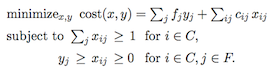## neal young / Young15Nearly

• working paper(2016)We describe the first nearly linear-time approximation algorithms for explicitly given mixed packing/covering linear programs, and for (non-metric) fractional facility location. We also describe the first parallel algorithms requiring only near-linear total work and finishing in polylog time. The algorithms compute $$(1+\epsilon)$$-approximate solutions in time (and work) $$\tilde O(N/\epsilon^2)$$, where $$N$$ is the number of non-zeros in the constraint matrix. For facility location, $$N$$ is the number of eligible client/facility pairs.